International
Tables for
Crystallography
Volume A
Space-group symmetry
Edited by M. I. Aroyo

International Tables for Crystallography (2016). Vol. A, ch. 3.1, pp. 707-708

Section 3.1.2.4. Example of Delaunay reduction and standardization of the basis

H. Burzlaffa and H. Zimmermannb

3.1.2.4. Example of Delaunay reduction and standardization of the basis

| top | pdf |

Let the basis B = (b1, b2, b3) given by the scalar products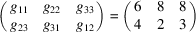or by b1 = 2.449 (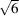), b2 = b3 = 2.828 (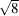) (in arbitrary units), β23 = 60° (cos β23 = ½), β13 = 73.22° (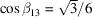), β12 = 64.34° (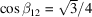).

The aim is to find a standardized basis of shortest lattice vectors using Delaunay reduction. This example, given by B. Gruber (cf. Burzlaff & Zimmermann, 1985), shows the standardization problems remaining after the reduction.

The general reduction step can be described using Selling four flats. The corners are designated by the vectors a, b, c, d = −abc. The edges are marked by the scalar products among these vectors. If positive scalar products can be found, choose the largest: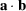(indicated as ab in Fig. 3.1.2.2a). The reduction transformation is: aD = a, bD = −b, cD = c + b, dD = d + b (see Fig. 3.1.2.2a). In this example, this results in the Selling four flat shown in Fig. 3.1.2.2(b). The next step, shown in Fig. 3.1.2.2(c), uses the (maximal) positive scalar product for further reduction. Finally, using b2 + b3 + b4 = −b1 we get the result shown in Fig. 3.1.2.2(d).Figure 3.1.2.2 | top | pdf |Delaunay reduction of Gruber's example (cf. Section 3.1.2.4). The edges of Selling tetrahedra are labelled by the scalar products of the vectors which designate the corners of the tetrahedra.

The complete procedure can be expressed in a table, as shown in Table 3.1.2.4. Each pair of lines contains the starting basis and its scalar products before transformation as the first line, and then the transformed scalar products and the Delaunay basis after transformation below. In our case, four transformation steps are necessary. The result is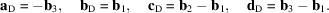The final Selling tetrahedron shows that the Dirichlet domain belongs to Voronoi type 1. It fulfils no symmetry condition and thus corresponds to an anorthic (triclinic) lattice.

 Table 3.1.2.4| top | pdf | Delaunay reduction for Gruber's example
b2 b3 b1 b4 +4 +3 −15 +2 14 −11
−4 +7 −11 −10 +6 −15 b2 b3 b1 + b3 b4 + b3
b1 + b3 b2 b3 b3 + b4 +7 −10 −15 −4 −11 +6
−7 −3 −8 −4 +3 −1 b1 + b3 b2 b2b3 b2 + b3 + b4
b1 b2 b2b3 b1 + b3 +3 −1 −8 −4 −7 −3
−3 +2 −5 −4 −1 −6 b1 b2 b3 b1b2 + b3
b3 b1 b2 b1b2 + b3 +2 −4 −6 −3 −5 −1
−2 −2 −4 −3 −1 −3 b3 b1 b2b1 b3b1

For further standardization we consider the Delaunay set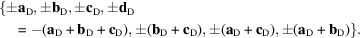All bases of shortest lattice vectors (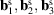) can be found: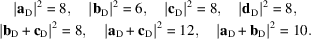Any basis of shortest lattice vectors contains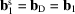. For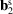the vectors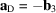,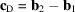,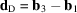and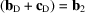are possible.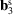can only be chosen from these vectors such that a linear independent triplet results.

The resulting five choices are given in Table 3.1.2.5. Any case corresponds to eight combinations of signs for the three basis vectors. The principle of the `homogenous corner' (i.e., there is always a pair of opposite corners of the corresponding cell where all angles are either non-acute or all three are acute) selects one of the bases in each case, thus five different bases remain. For the final choice the surfaces of the corresponding cells are given.

 Table 3.1.2.5| top | pdf | Discussion of Gruber's example using the cell surface
No.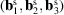Homogenous cornerSurface (surface units)
1 (+bD, +aD, +cD) Non-acute 41.25
2 (+bD, +aD, +dD) Non-acute 40.83
3 (+bD, −aD, bD + cD) Acute 39.61
4 (+bD, +cD, +dD) Non-acute 41.03
5 (+bD, −dD, bD + cD) Acute 40.06

The maximal surface has cell No. 1 with the metrical parameters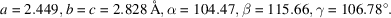A last possibility for the standardization is the interchange of b and c with inversion of all basis vectors. In this way the sequence of β and γ can be interchanged: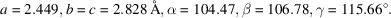References

Burzlaff, H. & Zimmermann, H. (1985). On the metrical properties of lattices. Z. Kristallogr. 170, 247–262.